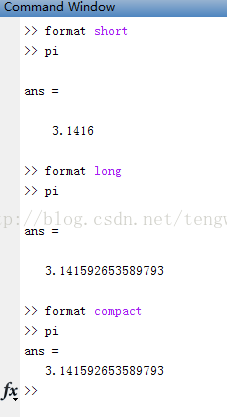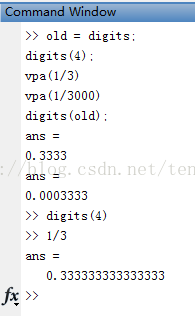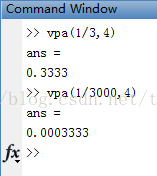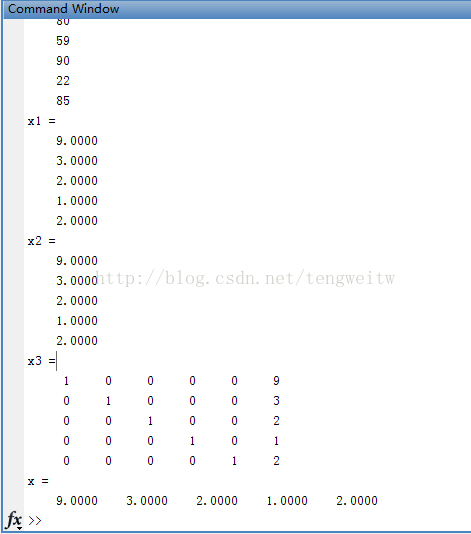0%

## 误差的来源

• 模型误差：数学模型与实际问题之间的误差
• 观测误差：测量数据与实际数据的误差
• 方法误差：数学模型的精确解与数值方法得到的数值解之间的误差：例如
• 舍入误差：对数据进行四舍五入后产生的误差

## 减少误差的几种方法

​ 现在，我们一般用计算机解决计算问题，使用最多的是Matlab软件。对实际问题进行数学建模时，可能存在模型误差，对数学模型进行数值求解时，我们使用的方法可能产生方法误差，我们输入计算机的数据一般是有测量误差的，计算机在运算过程的每一步又会产生舍入误差（十进制转化为二进制时可能产生舍入误差）。由此看来，解决一个问题，基本上会有以上四种四种误差。记得高中物理老师说过，错误是可以避免的，误差是不可避免的，我们只可以减少误差。下面我们就来介绍减少误差的几种方法：

• 避免两个相近的数相减
比如: 当$x=10003$时，计算$\sqrt{x+1}-\sqrt{x}$的近似值。
如果使用$6$位十进制浮点运算，运算时取$6$位有效数字，结果如下：

结果只有一位有效数字，与之前相比，损失了$5$位有效数字。

如果使用下面的方法：

则结果有$6$位有效数字，与精确值$0.00499912523117984\cdots$较为接近。

• 防止重要的小数被大数吃掉
比如: 已知$x=3\times 10^{12},y=7,z=-3\times 10^{12}$,求$x+y+z$
如果按照$(x+y)+z$的顺序来求的话，由于$x$远大于$y$，在计算机中可能导致$x+y=x$的情况，因此我们可以按照$(x+z)+y$的顺序计算得到正确的结果。

• 避免除数的绝对值远小于被除数的绝对值
比如：用消去法解线性方程组

这个方程组的正确解为：

对于上述方程组，当我们将第一个方程除以$0.00003$减去第二个方程时，可以得到下面的化简和计算结果：

显然上述结果严重失真，产生了很大的误差。这就是由于除数的绝对值远小于被除数的绝对值造成的。为了避免上述情况，我们可以用第二个方程消去第一个方程中的$x_1$，即第二个方程乘以$0.00003$减去第一个方程，得到如下表达式和结果：

将结果与正确解相比发现，这是一组相当好的近似解。

• 注意算法的稳定性
所谓算法的稳定性是指，一个算法如果输入数据有误差，而在计算过程中误差不增长，那么称此算法是数值稳定的。

#### 精度控制

• format digits vpa函数的使用
• format只用来控制显示精度的，并不控制计算精度，digits用来控制计算精度，vpa也是控制计算精度。
• digits必须与vpa配合使用，单独不起作用。
• vpa可单独控制计算精度

具体操作如下图：

1. ​ format的操作2. ​ digits的操作3. ​ vpa的操作#### 解非齐次线性方程组

• 求逆矩阵法

• 矩阵左除法

• 初等行变换

• 卡莱姆法则